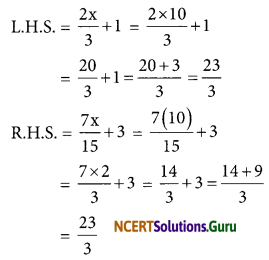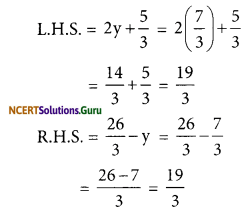# NCERT Solutions for Class 8 Maths Chapter 2 Linear Equations in One Variable Ex 2.3

These NCERT Solutions for Class 8 Maths Chapter 2 Linear Equations in One Variable Ex 2.3 Questions and Answers are prepared by our highly skilled subject experts.

## NCERT Solutions for Class 8 Maths Chapter 2 Linear Equations in One Variable Exercise 2.3

Solve the following equations and check your results.

Question 1.
3x = 2x + 18
Solution:
3x = 2x + 18
Transposing 2x from RHS to L.H.S, we get
3x – 2x = 18
x = 18

Check:
Put x = 18 in L.H.S. and R.H.S. of the equation.
L.H.S. = 3x = 3 × 18 = 54
R.H.S. = 2x + 18 = 2(18) + 18 = 36 +18 = 54
∴ L.H.S. = R.H.SQuestion 2.
5t – 3 = 3t – 5
Solution:
5t – 3 = 3t – 5
Transposing (-3) to R.H.S. and 3t to L.H.S., we get
5t – 3t = -5 + 3
2t = -2
Dividing both sides by 2, we get
$$\frac{2 t}{2}=\frac{-2}{2}$$
t = -1

Check:
Put t = -1 in L.H.S. and RHS of the equation
L.H.S. = 5t – 3 = 5(-1) – 3 = -5 – 3 = -8
R.H.S. = 3t – 5 = 3(-1) – 5 = -3 – 5 = -8
Hence, L.H.S. = R.H.S.

Question 3.
5x + 9 = 5 + 3x
Solution:
5x + 9 = 5 + 3x
Transposing 9 to R.H.S. and 3x to L.H.S.
5x – 3x = 5 – 9
2x = -4
Dividing both sides by 2, we get
$$\frac{2 x}{2}=\frac{-4}{2}$$
x = -2

Check:
Put x = -2 in L.H.S. and R.H.S. of the equation
L.H.S. = 5x + 9
= 5 (-2) + 9
= -10 + 9
= -1
R.H.S. = 5 + 3x
= 5 + 3(-2)
= 5 – 6 = -1
Hence, L.H.S. = R.H.S.Question 4.
4z + 3 = 6 + 2z
Solution:
4z + 3 = 6 + 2z
Transposing 3 to R.H.S. and 2z to LHS
4z – 2z = 6 – 3
2z = 3
Dividing both sides by 2, we get
$$\frac{2 z}{2}=\frac{3}{2}$$
z = $$\frac{3}{2}$$

Check:
Put z = $$\frac{3}{2}$$ in L.H.S. and R.H.S of the equation
L.H.S. = 4z + 3
= 4$$\left(\frac{3}{2}\right)$$ + 3
= 2(3) + 3
= 6 + 3
= 9
R.H.S = 6 + 2z
= 6 + 2$$\left(\frac{3}{2}\right)$$
= 6 + 3
= 9
Hence, L.H.S. = R.H.S.

Question 5.
2x – 1 = 14 – x
Solution:
2x – 1 = 14 – x
Transposing -1 to RHS and -x to L.H.S.
2x + x = 14 + 1
3x = 15
Dividing both sides by 3, we get
$$\frac{3 x}{3}=\frac{15}{3}$$
x = 5

Check:
Put x = 5 in LHS and RHS of the equation
LHS = 2x – 1
= 2(5) – 1
= 10 – 1
= 9
R.H.S = 14 – x
= 14 – 5
= 9
Hence, L.H.S. = R.H.S.Question 6.
8x + 4 = 3(x – 1) + 7
Solution:
8x + 4 = 3(x – 1) + 7
8x + 4 = 3x – 3 + 7
8x + 4 = 3x + 4
Transposing 4 to R.H.S. and 3x to LHS, we get
8x – 3x = 4 – 4
5x = 0
Dividing both sides by 5
$$\frac{5 x}{5}=\frac{0}{5}$$
x = 0

Check:
Put x = 0 in L.H.S. and R.H.S. of the equation
L.H.S. = 8x + 4
= 8(0) + 4
= 4
R.H.S = 3(x – 1) + 7
= 3(0 – 1) + 7
= -3 + 7
= 4
Hence, L.H.S. = R.H.S.

Question 7.
x = $$\frac{4}{5}$$ (x + 10)
Solution:
x = $$\frac{4}{5}$$ (x + 10)
Multiplying both sides by 5, we get
5x = 5 × $$\frac{4}{5}$$ (x + 10)
5x = 4(x + 10)
5x = 4x + 40
Transposing 4x to L.H.S.
5x – 4x = 40
x = 40

Check:
Put x = 40 in L.H.S. and R.H.S. of the equation
L.H.S. = x = 40
R.H.S. = $$\frac{4}{5}$$ (x + 10)
= $$\frac{4}{5}$$ (40 + 10)
= $$\frac{4}{5}$$ x 50
= 4 × 10
= 40
Hence, L.H.S. = R.H.SQuestion 8.
$$\frac{2 x}{3}+1=\frac{7 x}{15}+3$$
Solution:
$$\frac{2 x}{3}+1=\frac{7 x}{15}+3$$
Transposing 1 to R.H.S. and $$\frac{7 \mathrm{x}}{15}$$ to L.H.S.
$$\frac{2 x}{3}-\frac{7 x}{15}=3-1$$
$$\frac{10 x-7 x}{15}$$ = 2
$$\frac{3 x}{15}$$ = 2
Multiplying both sides by 15, we get
$$\frac{3 x}{15}$$ × 15 = 2 × 15
3x = 30
Dividing both sides by 3
$$\frac{3 x}{3}=\frac{30}{3}$$
x = 10

Check:
Put x = 10 in L.H.S. and R.H.S. of the equationHence, L.H.S. = R.H.S.

Question 9.
$$2 y+\frac{5}{3}=\frac{26}{3}-y$$
Solution:
$$2 y+\frac{5}{3}=\frac{26}{3}-y$$
Transposing $$\frac{5}{3}$$ to R.H.S. and -y to L.H.S.
2y + y = $$\frac{26}{3}-\frac{5}{3}$$
3y = $$\frac{26-5}{3}$$
3y = $$\frac{21}{3}$$ = 7
Divide both sides by 3, we get
$$\frac{3 y}{3}=\frac{7}{3}$$
y = $$\frac{7}{3}$$

Check:
Put y = $$\frac{7}{3}$$ in .L.H.S. and R.H.S of the equationHence, L.H.S. = R.H.SQuestion 10.
3m = 5m – $$\frac{8}{5}$$
Solution:
3m = 5m – $$\frac{8}{5}$$
Transposing 5m to L.H.S. we get
3m – 5m = $$\frac{-8}{5}$$
-2m = $$\frac{-8}{5}$$
Dividing both sides by -2, we get
$$\frac{-2 m}{-2}=\frac{-8}{5} \div(-2)$$
m = $$\frac{8}{10}=\frac{4}{5}$$

Check:
Put m = $$\frac{4}{5}$$ in L.H.S. and R.H.S. of the equationHence, L.H.S = R.H.S

error: Content is protected !!